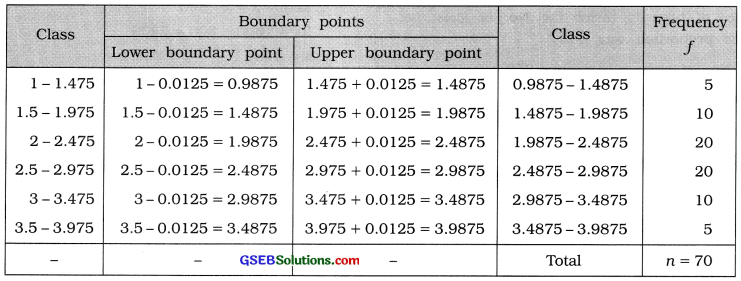# GSEB Solutions Class 11 Statistics Chapter 2 Presentation of Data Ex 2.1

Gujarat Board Statistics Class 11 GSEB Solutions Chapter 2 Presentation of Data Ex 2.1 Textbook Exercise Questions and Answers.

## Gujarat Board Textbook Solutions Class 11 Statistics Chapter 2 Presentation of Data Ex 2.1

Question 1.
The data regarding the number of children of 50 families residing in a certain area are given below. Prepare an appropriate frequency distribution.Here, the number of children in a family is discrete variable. Therefore we will obtain discrete frequency distribution. In the frequency distribution x = No. of children, f = No. of families. In the given data, the minimum value of x is 0 and the maximum value is 3. Hence the discrete frequency distribution of the number of children is obtained as follows :

Discrete frequency distribution showing the number of children in 50 families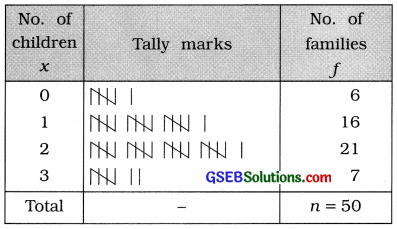Question 2.
The ages (in full years) of 60 employees working in an office are registered as follows.
Prepare a frequency distribution by taking class length as 5 from this information.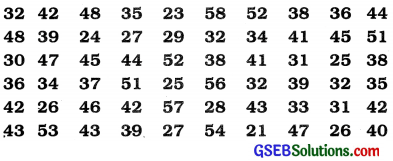In the given data, the minimum age of an employee is 21 years and the maximum age is 58 years. Class length 5 is given. Therefore the lower limit (which is multiple of 5) of the initial class we will take 20 and the upper limit (which is also multiple of 5) of the last class we will take 60. Thus, the initial class and the last class of the frequency distribution will be respectively 20 – 25 and 55-60.

Continuous frequency distribution showing the age of 60 employees of an officeQuestion 3.
The data regarding the number of mobile phones produced during last 60 days by a mobile phone manufacturing company is given below. Distribute it into 10 classes.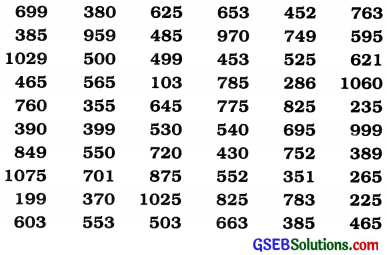Obtain ‘less than’ and ‘more than’ type cumulative frequency distribution from it.
In the given data, the minimum production of mobile is 188 and the maximum is 1075. Therefore, range of data R = 1075 – 188 = 887 mobiles. The data is to be divided in 10 classes. Therefore, k = 10 range
Now, class length c = $$\frac{\text { range }}{\text { no. } \text { of classes }}=\frac{\mathrm{R}}{k}$$
∴ c = $$\frac{887}{10}$$ = 88.7 ≈ 89
If c = 89, then ck = 89 x 10 = 890, which is greater than R, i.e., ck > R. Hence, we can take c = 89. But, usually the value of c should be taken which is multiple of 10. Hence, c = 100 is taken.

Here, the range of data is large. Hence, it is advisable to prepare inclusive frequency distribution, c = 100. therefore the initial class which includes the lowest observation 188 will be 100-199 and the last class which includes the highest observation 1075 will be 1000-1099. Thus, inclusive continuous frequency distribution is obtained as follows :

Inclusive continuous frequency distribution, showing the production of mobiles during 60 days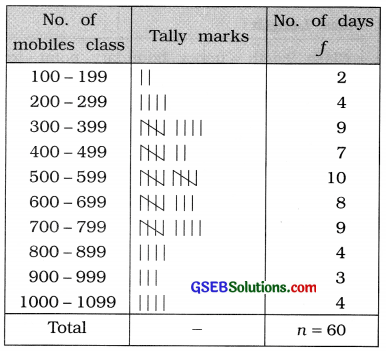Explanation: For ‘less than’ and ‘more than’, cumulative frequency distribution, we have to obtain upper class boundary point and lower class boundary point of the classes respectively.
For initial class 100-199,
Lower class boundary point = $$\frac{99+100}{2}$$ = 99.5;
Upper class boundary point = $$\frac{199+200}{2}$$ = 199.5]
[Alternative method: The upper limit of the class 100-199 is 199 and the lower limit of immediate class 200 – 299 is 200. So their difference is (200 – 199 = ) 1. Dividing this difference by 2, we get($$\frac{1}{2}$$ = ) 0.5. Subtracting
this difference from the lower limit of each class and adding it to the upper limit of each class, we get lower boundary point and upper boundary point respectively for each class.]

Less than’ type cumulative frequency distribution‘More than’ type cumulative frequency distribution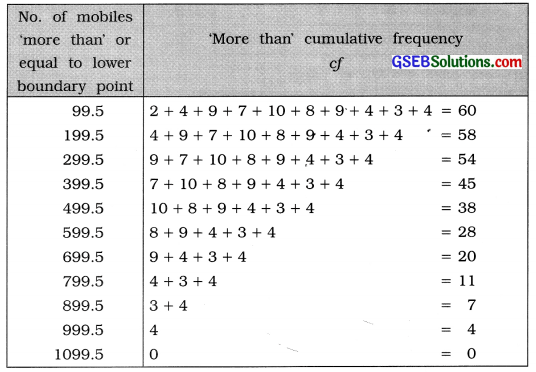Question 4.
Rewrite the following frequency distribution by stating class length and Mid Value of each class :Given frequency distribution is inclusive type. Here, the difference between the upper limit of each class and the lower limit of its immediate adjoining class is 1. Subtracting $$\frac{1}{2}$$ = 0.5 from the lower limit of each class and adding it to the upper limit of each class, we get lower boundary point and upper boundary point respectively for each class, which is shown in the table on page 28.Question 5.
From the following frequency distribution, obtain ‘less than’ and ‘more than’ type cumulative frequency distributions :x = No. of errors per page, f = No. of pagesQuestion 6.
Obtain an inclusive continuous frequency distribution from the following data: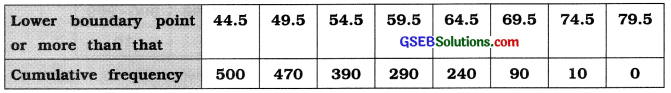Given data is ‘more than’ type cumulative frequency distribution.
∴ Class length = Difference between the adjoining lower boundary points = 54.5 – 49.5 = 5
Now, upper boundary point of the class = lower boundary point + class length
For initial class lower boundary point = 44.5
∴ initial class is 44.5 – 49.5.

For inclusive continuous frequency distribution lower limit of initial class = 44.5 + 0.5 = 45 and upper limit of initial class = 49.5 – 0.5 = 49.
Thus, for given data the initial class in inclusive form, we get 45-49.
In this manner, we will obtain the class for each lower boundary point.
We will find the frequency of each class from the given ‘more than’ cumulative frequency as follows :

Frequency of a class = (‘more than’ cumulative frequency of a class) – (‘more than cumulative frequency of immediate following class)

Frequency of class for 44.5 = (‘more than’ cumulative frequency of a class for 44.5) – (‘more than cumulative frequency of immediate following class for 49.5)
= (500 – 470) = 30

In this manner we will obtain the frequency for the rest of classes.
For the given data, we get the inclusive continuous frequency distribution as follows :

 Lower Boundary point or more More than’ cumulative frequency Cf Class Frequency f 44.5 500 45-49 500 – 470 = 30 49.5 470 50-54 470 – 390 = 80 54.5 390 55-59           . 390 – 290 = 100 59.5 290 60-64 290 – 240 = 50 64.5 240 65-69 240 – 90 = 150 69.5 90 70-74 90 – 10 = 80 74.5 10 75-79 10 – 0 = 10 79.5 0 80-84 = 0 – – Total n = 500Question 7.
Obtain an exclusive continuous frequency distribution from the following data: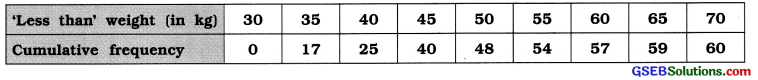Given data is ‘less than’ type cumulative frequency distribution.
Class length = Difference between two adjoining upper boundary points
= 35 – 30 = 5
Now, lower boundary point of a class = upper boundary point – class length
∴ for initial class, lower boundary point = 30 – 5 = 25 and we get initial class 25 – 30.

In this manner we will obtain class for each upper boundary point.
We find the frequency of a class as follows :
Frequency of a class = (‘less than ‘ cumulative frequency of a class) – (‘less than’ cumulative frequency of preceeding class)
Class frequency for upper boundary point 35 = 17 – 0 = 17
In this manner, we will obtain the class frequency for each upper boundary point.

For the given data exclusive continuous frequency distribution is obtained as follows:

 Weight ‘less than’ in kg ‘Less than’ cumulative frequency cf Weight(in kg) Frequency f 30 0 25-30 = 0 35 17 30-35 17- 0 = 17 40 25 35-40 25 – 17 = 8 45 40 40-45 40 – 25 = 15 50 48 45-50 48 – 40 = 8 55 54 50-55 54 – 48 = 6 60 57 55-60 57 – 54 = 3 65 59 60-65 59 – 57 = 2 70 60 65-70 60 – 59 = 1 – – Total n = 60

Question 8.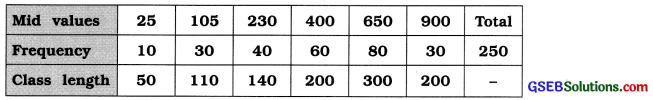Obtain the original frequency distribution.
In the given data, mid values and class length are given. We will find the lower limit and upper limit of a class as follows :
Lower limit = mid value – $$\frac{\text { class length }}{2}$$
Upper limit = mid value + $$\frac{\text { class length }}{2}$$
For mid value 25, lower limit of the class = 25 – $$\frac{50}{2}$$ = 25 – 25 = 0 and
upper limit of the class = 25 + $$\frac{50}{2}$$ = 25 + 25 = 50
∴ For mid value 25, we get the class 0 – 50.

In this manner, we will obtain the class for each mid value.
Thus, original frequency distribution is obtained as follows:Question 9.
Information regarding the number of accidents in a city during a year is as under. Find the inclusive continuous frequency distribution from it.In given data, mid values of class and their frequency are given.
The difference between two adjoining mid values is 10.
∴ class length =10[Note : For inclusive class : Lower boundary point = Lower limit + 0.5; Upper boundary point = Upper limit – 0.5]Question 10.
From the following data, obtain class boundary points from the class limits and write the frequency distribution: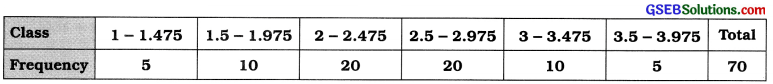Subtracting $$\frac{0.025}{2}$$ = 0.0125 from the lower limit of the class and adding it to the upper limit, we get lower boundary point and upper boundary point respectively of the classes.